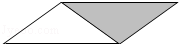首页 / 小学数学 / 试卷选题

# 苏教版五年级（上）期末数学

﹣50元表示支出50元，那么+100元表示　　　　　　；零上5℃通常记作　　　　　　，低于海平面120米通常记作

• 题型：未知
• 难度：未知• 题型：未知
• 难度：未知

5个一和4个百分之一组成的数是　　　　　　；10.70里面有一个十和　　　　　　个十分之一．

• 题型：未知
• 难度：未知

2003年我国粮食产量达到430670000吨，改写成以万吨作单位的数是　　　　　　，省略亿后面的尾数是

• 题型：未知
• 难度：未知

）如图平行四边形的面积是32平方厘米，涂色部分的面积是　　　　　　平方厘米．• 题型：未知
• 难度：未知

• 题型：未知
• 难度：未知

3.4×2.9=　　　　　　；
0.34×0.29=
9.86÷0.29=　　　　　　；
9.86÷0.034=

• 题型：未知
• 难度：未知

 0.7　　　　　　0.56 1.060　　　　　　1.06 4.8×0.99　　　　　　4.8 9.5÷0.88　　　　　　9.5．

• 题型：未知
• 难度：未知

120厘米=　　　　　　米；
2.5吨=　　　　　　千克；
0.5公顷=　　　　　　平方米；
10000000平方米=　　　　　　平方千米．

• 题型：未知
• 难度：未知

• 题型：未知
• 难度：未知

• 题型：未知
• 难度：未知

3个面积1平方分米的正方形拼成一个长方形，在长方形里画一个梯形（如图），这个梯形的面积是多少？（　　）A．4平方分米      B．3平方分米      C．2平方分米

• 题型：未知
• 难度：未知

A．3.06  B．3.07  C．3.09

• 题型：未知
• 难度：未知A．＞ B．＜ C．= D．无法判定

• 题型：未知
• 难度：未知

A．米     B．平方米     C．公顷

• 题型：未知
• 难度：未知

A．﹣1   B．2       C．3

• 题型：未知
• 难度：未知

 6+2.4= 0.3×0.2= 0.55﹣0.5= 4.8÷6= 6.6×0.1= 0.5×2.4= 1﹣0.2= 4.6+5.4= 0.2÷0.01= 0.8×100= 6×0.01= 5.5÷10=

• 题型：未知
• 难度：未知

 9.73+0.27= 6﹣1.55= 3.05×0.66= 22.5÷0.25=

• 题型：未知
• 难度：未知

 5.27﹣1.98﹣0.02 3.2×5.7+6.8×5.7 2.5×6.8×0.4 5.6﹣36÷30 0.72+9.6÷3.2 7.75×[20÷（3.24﹣3.04）]．

• 题型：未知
• 难度：未知• 题型：未知
• 难度：未知• 题型：未知
• 难度：未知• 题型：未知
• 难度：未知

（1）从22日至24日，最高气温下降了　　　　　　℃．
（2）温差（日最高气温和最低气温的差）最大的是　　　　　　日，温差最小的是　　　　　　日．
（3）估一估，哪两天的平均气温可能低于22°C？　　　　　　日和　　　　　　日．• 题型：未知
• 难度：未知• 题型：未知
• 难度：未知

• 题型：未知
• 难度：未知

• 题型：未知
• 难度：未知• 题型：未知
• 难度：未知• 题型：未知
• 难度：未知

 名称 数量 单价 总价 面 粉 25千克 元 70.00元 色拉油 升 8.00元 元 合计金额：壹佰叁拾贰元肆角整¥132.40元

• 题型：未知
• 难度：未知